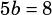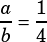Test 2 Section 3 Number 20 please!

When a system of linear equations has an infinite number of solutions, what that really means is that both linear equations produce the same line!

Because we know the equations are equivalent, let’s multiply the top equation by 5 on both sides so that we have both equations equaling 60.Since the equations are equivalent, we know that the corresponding coefficients are equal:and. From that, we can conclude that, which of course simplifies to.